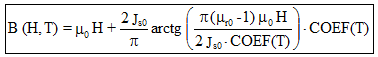# Analytic saturation curve * exponential function of T

## Presentation

This model defines a B(H) behavior law:

• nonlinear (taking the saturation into consideration)
• for an isotropic material
• through a value of the saturation magnetic polarization Js which decreases in an exponential way when the temperature increases

## Mathematical model

This model is a combination of a straight line and an arc tangent curve. In this model, the saturation magnetic polarization Js decreases in an exponential way when the temperature increases (Js0 is multiplied by the value of a temperature coefficient COEF(T)).

The corresponding mathematical formula is written:where:

• μ0 is the permeability of vacuum; μ0 = 4 π 10-7 [H/m]
• μr0 is the relative permeability of material (at the origin) for T = 0°C
• Js0 is the saturation magnetic polarization for T = 0°C [Tesla]
• COEF(T) is the previously described temperature coefficient

The shape of B(H) curve is presented in the figure below.## Example

An example of a B(H) curve dependent on T is presented in the figure below.

It is an analytic saturation curve * exponential function of T with:

Tcurie = 720°, C = 100°C